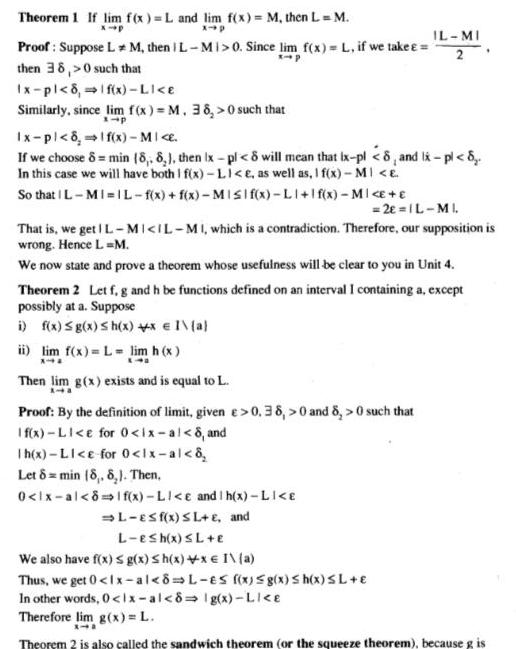Question:

# Theorem 1 If lim f x L and lim f x M then L M x p x p IL MI

Last updated: 9/17/2023Theorem 1 If lim f x L and lim f x M then L M x p x p IL MI Proof Suppose L M then IL MI 0 Since lim f x L if we take 2 then 38 0 such that x p Ix pl 8 f x LI E Similarly since lim f x M 38 0 such that x p Ix pl 8 If x MI e If we choose 8 min 8 8 then Ix pl 8 will mean that ix pl 8 and lx pl 8 In this case we will have both I f x LI e as well as I f x MI e So that IL MI L f x f x MISI f x LI f x MI E 2 IL MI That is we get L MI IL MI which is a contradiction Therefore our supposition is wrong Hence L M We now state and prove a theorem whose usefulness will be clear to you in Unit 4 Theorem 2 Let f g and h be functions defined on an interval I containing a except possibly at a Suppose i f x g x h x x I a ii lim f x L lim h x X 48 Then lim g x exists and is equal to L 243 Proof By the definition of limit given e 0 38 0 and 8 0 such that If x LI e for 0 ix al 8 and Ih x LI e for 0 lx al 8 Let 8 min 8 8 Then 0 lx al 8 lf x LI e and I h x LI E L ESf x SL E and L esh x SL E We also have f x g x Sh x xE I a Thus we get 0 1x al 8 L ES f x g x h x L In other words 0 lx al 8 Ig x Li e Therefore lim g x L 113 Theorem 2 is also called the sandwich theorem or the squeeze theorem because g is